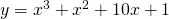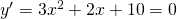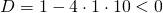# Solution assignment 02 Optimizing f(x)

### Assignment 2

Verify whether the graph of the function has extremes:and if so, whether it is a maximum of a minimum.

### Solution

We find candidates for a maximum or minimum by solving the equation:This quadratic equation has no solutions because the discriminant:The graph has no (local) extremes nor a horizontal inflection point.

0